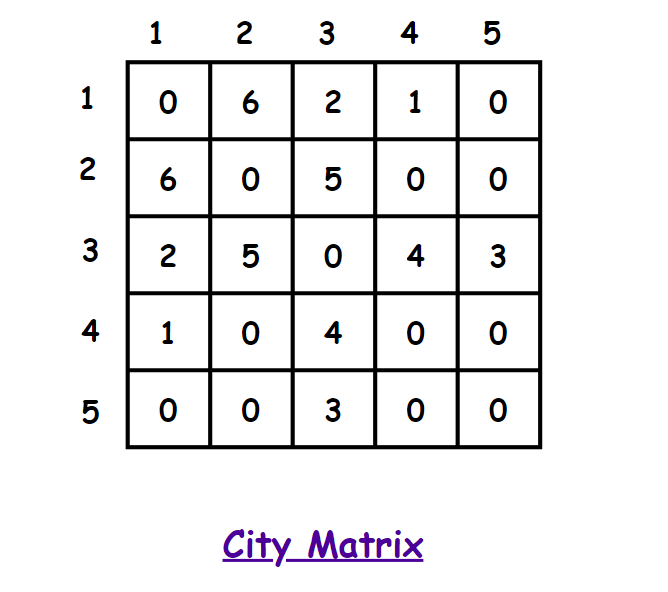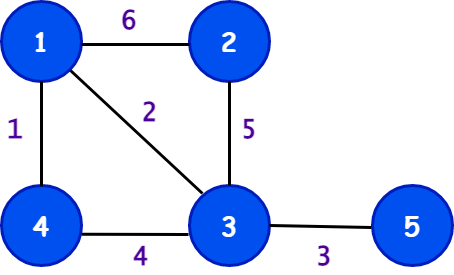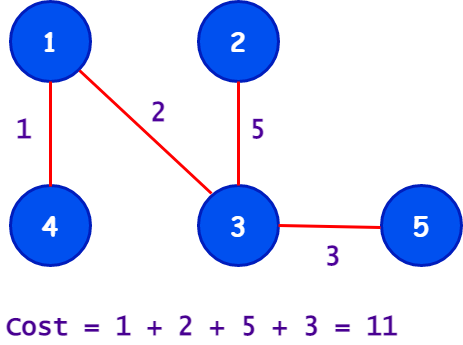# Minimum cost to connect all cities

## Introduction

In this article, we will discuss a very interesting problem based on the application of graph theory. We will solve the problem in one of the most intuitive ways and build the solution step by step, similar to how you are expected to solve an unknown problem in a coding interview.

You will also get to know the detailed analysis of time and space complexities, which will help you develop the complexity analysis of other questions too.

Let’s get started with the problem statement.

## Problem Statement

There are n cities and roads in between some of the cities. Somehow all the roads are damaged simultaneously. We have to repair the roads to connect the cities again. There is a fixed cost to repair a particular road.

Input is in matrix(city) form, if city[i][j] = 0 then there is not any road between city i and city j, if city[i][j] = a > 0 then the cost to rebuild the path between city i and city j is a.

Find out the minimum cost to connect all the cities by repairing roads.

### Example

Let’s take some examples to understand the goal of this problem.

Input

n=5

matrix city[n][n] is given as -Output

``11``

Explanation

If we consider the cities as nodes and the roads between them as edges of a graph, then we will obtain a weighted graph as shown below -Among all possible ways, the most optimal path connecting all the cities to get the minimum cost is:So, we obtain the minimum cost by repairing the roads connecting the cities 1-4, 1-3, 3-2, and 3-5.

Please try to solve this problem on your own before moving on to further discussion here - Connecting Cities With Minimum Cost

## How to approach the problem?

The most important step towards approaching the problem is to first understand the problem. So, here you have to find the minimum cost to connect all the cities.

What do you understand by the problem statement? 💡

Connecting all cities implies that there should exist at least one path between every two cities. That is, you should be able to travel from any one city to any other city through a road connecting them.

You might think that I have n cities and some roads to repair. Now, I just need to connect the cities by repairing the roads such that all cities are connected. Well, this sounds easy. I will repair all the roads and check if all cities are connected.

Does this work?

Well, this solves just a part of the problem.

There are two goals to achieve in this problem -

• Connect all cities
• The cost of connecting them should be minimum

Do we have a standard concept in graph theory that achieves the above two requirements?

The great news is, Yes🤩. Can you remember?

If yes, then well done!! You must have been practising the graph theory concepts by heart.

If not, it's never too late to learn an important concept 😎. Before moving further to make the most out of this blog, you should consider reading this beginner-friendly article: Introduction and Properties of Minimum Spanning Tree.

We know about Minimum Spanning Tree(MST) in a graph, and the problem in this blog is a direct application of MST.

Minimum Spanning Tree(MST) - A minimum spanning tree is nothing but a subset of a graph that connects all the vertices without forming a cycle and with minimum cost.

## Approach

In our case, vertices = cities and edges = roads.
Simply, we will apply the algorithm to find the MST of the graph given to us, which will be the required answer.

We will use Prim’s algorithm to find the MST.

It’s time 🕒 to see the approach step by step to make your understanding crystal clear:

• To find MST, first, we need to have a graph. The matrix city[][] represents the adjacency matrix of the graph where city[i][j] is zero if no path exists between city i and city j, and if city[i][j] is non-zero, then it denotes the cost to repair the road between them.

• Create a function prims() to find MST and pass the matrix city and number of cities n as parameters.

• In the function prims(), create three arrays of size n namely weight[], visited[] and parent[].

• Initialise the weights of all the nodes as INT_MAX and mark all nodes as not visited by initialising the visited[] array to false.

• Next, there will be total n nodes in the MST, so; we include the first node, i.e. node-0, by making weight=0 and parent=-1.

• To find the other n-1 nodes run a loop n-1 times and in each iteration, find the node having minimum weight and include it in the MST by making visited[minimum_node]=true and also update the weights of the neighbours of this node that have not been visited yet according to the following
condition - if city[a][b] < weight[b] where ‘a’ is the minimum_node and ‘b’ is its neighbour then make weight[b]=city[a][b] and parent[b]=a.

• After completing all the iterations, traverse the minimum spanning tree and its edge weights to compute the minimum cost, the required output.

Let’s see the code implementation along with the analysis of time and space complexity in the next section.

### C++ Implementation

``````/*C++ code to find minimum cost to connect all cities*/
#include <bits/stdc++.h>
using namespace std;

int findMinNode(int *weight, bool *visited, int n)
{
int minIndex = -1;
for (int i = 0; i < n; i++)
{
if (!visited[i] && (minIndex == -1 || weight[i] < weight[minIndex]))
{
minIndex = i;
}
}
return minIndex;
}

int primMST(vector<vector<int>> &city, int n)
{
int weight[n];
bool visited[n];
int parent[n];
for (int i = 0; i < n; i++)
{
weight[i] = INT_MAX;
visited[i] = false;
}
parent = -1;
weight = 0;
for (int i = 1; i <= n - 1; i++)
{
int minNode = findMinNode(weight, visited, n);
visited[minNode] = true;

for (int j = 0; j < n; j++)
{
if (city[minNode][j] > 0 && !visited[j])
{
if (city[minNode][j] < weight[j])
{
weight[j] = city[minNode][j];
parent[j] = minNode;
}
}
}
}
int ans = 0;
for (int i = 1; i < n; i++)
{
ans += weight[i];
}

return ans;
}

int main()
{
int n = 5;

vector<vector<int>> city = {
{0, 6, 2, 1, 0},
{6, 0, 5, 0, 0},
{2, 5, 0, 4, 3},
{1, 0, 4, 0, 0},
{0, 0, 3, 0, 0}};

int minCost = primMST(city, n);
cout << "The minimum cost to connect all cities = " << minCost << endl;
}``````

Output

``The minimum cost to connect all cities = 11``

### Java Implementation

``````import java.io.*;
import java.util.*;

class Solution
{

static int findMinNode(int[] weight, boolean[] visited, int n)
{
int minIndex = -1;
for (int i = 0; i < n; i++)
{
if (!visited[i] && (minIndex == -1 || weight[i] < weight[minIndex]))
{
minIndex = i;
}
}
return minIndex;
}

static int primMST(int city[][], int n)
{
int weight[]=new int[n];
boolean visited[]=new boolean[n];
int parent[]=new int [n];
for (int i = 0; i < n; i++)
{
weight[i] = Integer.MAX_VALUE;
visited[i] = false;
}
parent = -1;
weight = 0;
for (int i = 1; i <= n - 1; i++)
{
int minNode = findMinNode(weight, visited, n);
visited[minNode] = true;

for (int j = 0; j < n; j++)
{
if (city[minNode][j] > 0 && !visited[j])
{
if (city[minNode][j] < weight[j])
{
weight[j] = city[minNode][j];
parent[j] = minNode;
}
}
}
}
int ans = 0;
for (int i = 1; i < n; i++)
{
ans += weight[i];
}

return ans;
}

public static void main(String[] args) {
int n = 5;

int city[][] = {
{0, 6, 2, 1, 0},
{6, 0, 5, 0, 0},
{2, 5, 0, 4, 3},
{1, 0, 4, 0, 0},
{0, 0, 3, 0, 0}};

int minCost = primMST(city, n);
System.out.println("The minimum cost to connect all cities = "+minCost);

}
}``````

Output

``The minimum cost to connect all cities = 11``

### Python Implementation

``````
#  Function for determining the lowest valued node among nodes not yet included in MST.
def minnode(n, keyval, mstset):
mini = 999999999999
mini_index = None

# Find the lowest valued node by looping through all the values of the nodes that aren't yet included in MST.
for i in range(n):
if (mstset[i] == False and
keyval[i] < mini):
mini = keyval[i]
mini_index = i
return mini_index

# The MST as well as the cost of the MST are calculated using this function.

def findcost(n, city):

# The parent node of a given node is stored in this array.
parent = [None] * n

# Each node's key value is stored in an array.
keyval = [None] * n

# Boolean Array to hold bool values indicating whether or not a node is included in MST.
mstset = [None] * n

# Set all of the key values to infinite, and make sure that none of the nodes are in MST.
for i in range(n):
keyval[i] = 9999999999999
mstset[i] = False

# Begin looking for the MST at node 0.
# Because node 0 has no parent, set -1.
# a critical value or the cheapest way to get there
# The 0th node from the 0th node has a value of 0.
parent = -1
keyval = 0

# Find the rest n-1 nodes of MST.
for i in range(n - 1):

# First, determine the smallest node
# among the nodes that have not yet been included in MST.
u = minnode(n, keyval, mstset)

# Now the uth node is included in MST.
mstset[u] = True

# Update the values of u's neighbouring nodes that haven't yet been included in the MST.
for b in range(n):
if (city[u][b] and mstset[b] == False and
city[u][b] < keyval[b]):
keyval[b] = city[u][b]
parent[b] = u

# Calculate the cost by adding the MST edge values.
cost = 0
for i in range(1, n):
cost += city[parent[i]][i]
print(cost)

# Driver Code
if __name__ == '__main__':

# Input 1
n1 = 5
city1 = [[0, 6, 2, 1, 0],
[6, 0, 5, 0, 0],
[2, 5, 0, 4, 3],
[1, 0, 4, 0, 0],
[0, 0, 3, 0, 0]]
print("Minimum cost to connect all the cities is:",end=" ")
findcost(n1, city1)

#  Function for determining the lowest valued node among nodes not yet included in MST.
def minnode(n, keyval, mstset):
mini = 999999999999
mini_index = None

# Find the lowest valued node by looping through all the values of the nodes that aren't yet included in MST.
for i in range(n):
if (mstset[i] == False and
keyval[i] < mini):
mini = keyval[i]
mini_index = i
return mini_index

# The MST as well as the cost of the MST are calculated using this function.

def findcost(n, city):

# The parent node of a given node is stored in this array.
parent = [None] * n

# Each node's key value is stored in an array.
keyval = [None] * n

# Boolean Array to hold bool values indicating whether or not a node is included in MST.
mstset = [None] * n

# Set all of the key values to infinite, and make sure that none of the nodes are in MST.
for i in range(n):
keyval[i] = 9999999999999
mstset[i] = False

# Begin looking for the MST at node 0.
# Because node 0 has no parent, set -1.
# a critical value or the cheapest way to get there
# The 0th node from the 0th node has a value of 0.
parent = -1
keyval = 0

# Find the rest n-1 nodes of MST.
for i in range(n - 1):

# First, determine the smallest node
# among the nodes that have not yet been included in MST.
u = minnode(n, keyval, mstset)

# Now the uth node is included in MST.
mstset[u] = True

# Update the values of u's neighbouring nodes that haven't yet been included in the MST.
for b in range(n):
if (city[u][b] and mstset[b] == False and
city[u][b] < keyval[b]):
keyval[b] = city[u][b]
parent[b] = u

# Calculate the cost by adding the MST edge values.
cost = 0
for i in range(1, n):
cost += city[parent[i]][i]
print(cost)

# Driver Code
if __name__ == '__main__':

# Input 1
n1 = 5
city1 = [[0, 6, 2, 1, 0],
[6, 0, 5, 0, 0],
[2, 5, 0, 4, 3],
[1, 0, 4, 0, 0],
[0, 0, 3, 0, 0]]
findcost(n1, city1)``````

Output

``The minimum cost to connect all the cities = 11``

### Time Complexity

O(n2), where n=total number of cities.

In the function primMST, we have one loop of length n-1 to include the nodes in MST and in every iteration, it computes the node with minimum node, which takes O(n) time and also updates the value of its neighbouring nodes, which also takes O(n) time. So, effectively in every iteration the total time is - O(n) + O(n) = O(n) only.

And since we have total n-1 such iterations, so the total time complexity becomes (n-1)*O(n) = O(n2).

### Space Complexity

O(n), where n=total number of cities.

In the function primMST, we use three arrays of size n each. Hence the total space complexity is O(n)+O(n)+O(n) = O(n).

1. What is MST in Prims?
MST stands for Minimum Spanning Tree, which describes the subset of a graph containing all the vertices connected by edges such that the sum of the weights of edges in the subgraph is minimum.

2. Which one is better: Prims or Kruskal?
If the graph consists of lots of edges, i.e. for dense graphs, then Prim’s Algorithm is more efficient than Kruskal’s algorithm.

3. Where to practice the most important Graph Interview Questions?
You can practice the TOP GRAPHS INTERVIEW QUESTIONS on Codestudio.

## Key Takeaways

In this article, we discussed a problem based on the direct application of a minimum spanning tree.

In the question, it was not mentioned to use MST, but breaking down the problem statement into two goals, i.e. a) connect all cities and b) minimum cost, helped us to deduce that finding the MST can suffice both the requirements.

The graph was obtained by considering cities as vertices, roads as edges and the cost to repair the roads as weights of the edges.

Most of the problems based on graphs are not given straightforward, but it is twisted in terms of real-world problems, and you just need to read between the lines to solve the problem using existing graph techniques like BFS, DFS, MST etc.

Learn about Prims Algorithm- Minimum Spanning Tree (MST) from codestudio.

You can try solving the following problems based on finding minimum spanning tree - on Codestudio -

Are you planning to ace the interviews of reputed product-based companies like Amazon, Google, Microsoft, and more?

Attempt our Online Mock Test Series on CodeStudio now!